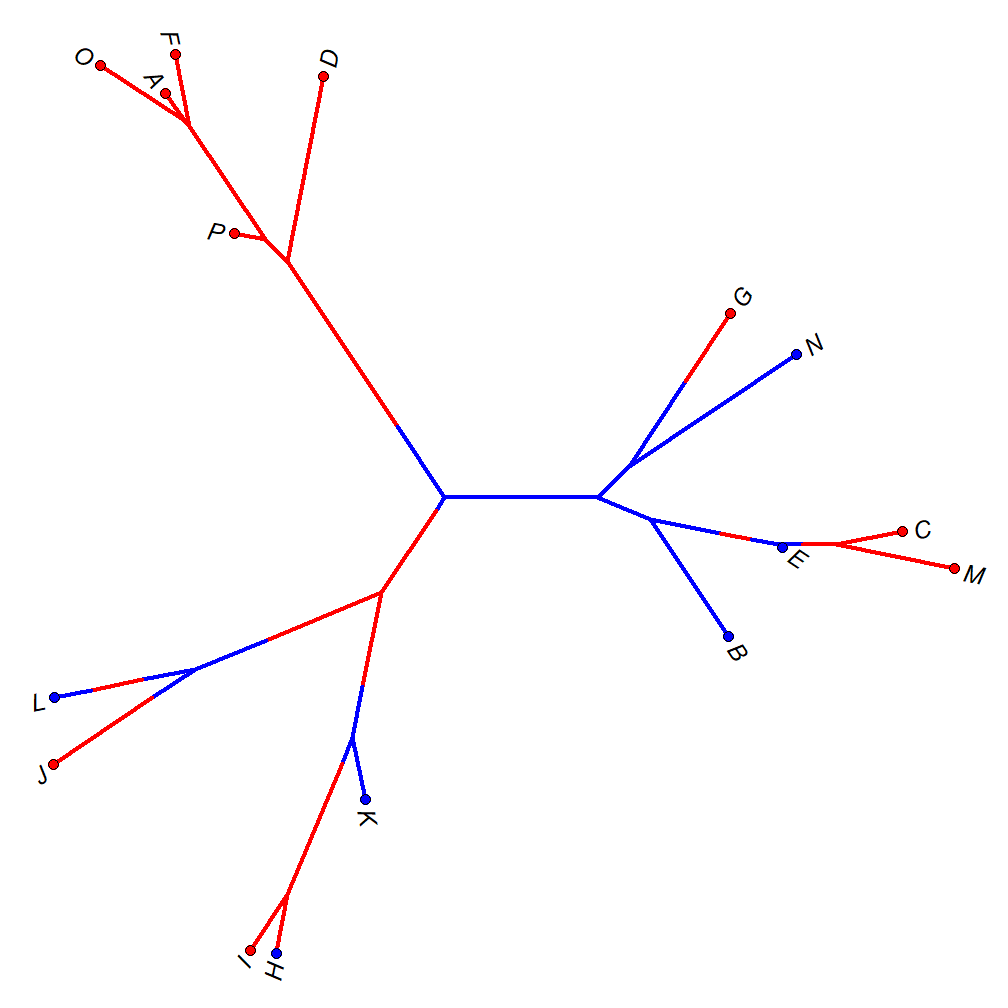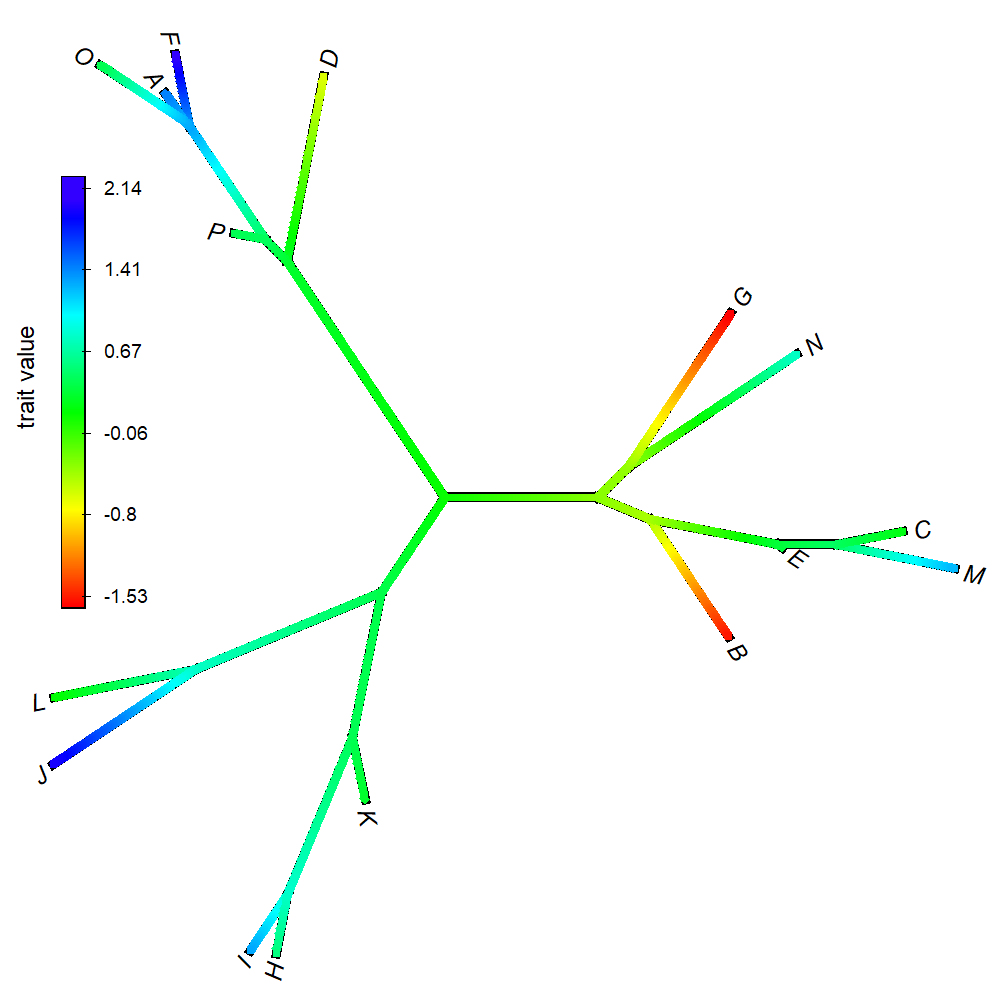## Thursday, October 18, 2018

### Plotting a `"simmap"` or `"contMap"` object in unrooted style

I haven't been posting to actively to this blog lately I have been busy with teaching and with my 'new' package learnPopGen as well as with the various shiny web interfaces that I have built for a number of the functions of the package.

Nonetheless, this morning for some reason I was thinking about the problem of plotting unrooted trees (which is actually much more complicated that plotting rooted trees in their various configurations, as discussed in Chapter 34 of Felsenstein 2004), and I suddenly realized that to plot trees with a mapped distrete character (objects of class `"simmap"`) or trees with a mapped continuous character (objects of class `"contMap"`) I could quite easily take advantage of `ape::plot.phylo` and simply project my tree on top of the coordinates of the unrooted tree plotted by `plot.phylo` in which this problem has already been solved.

This is what I mean.

``````library(phytools)
set.seed(777)
N<-16
tree<-rtree(n=N,tip.label=LETTERS[1:N])
Q<-matrix(c(-1,1,1,-1),2,2,dimnames=list(letters[1:2],letters[1:2]))
x<-sim.Mk(tree,Q)
x
``````
``````## L J I H K B E M C N G D F A O P
## a b b a a a a b b a b b b b b b
## Levels: a b
``````
``````map.tree<-make.simmap(tree,x)
``````
``````## make.simmap is sampling character histories conditioned on the transition matrix
##
## Q =
##           a         b
## a -1.038569  1.038569
## b  1.038569 -1.038569
## (estimated using likelihood);
## and (mean) root node prior probabilities
## pi =
##   a   b
## 0.5 0.5
``````
``````## Done.
``````
``````## here's where it gets interesting:
plot.phylo(map.tree,type="unrooted",lab4ut="axial",no.margin=TRUE,
label.offset=0.05)
obj<-get("last_plot.phylo",envir=.PlotPhyloEnv)
tip.pos<-matrix(c(obj\$xx[1:Ntip(map.tree)],obj\$yy[1:Ntip(map.tree)]),
Ntip(map.tree),2,dimnames=list(map.tree\$tip.label,NULL))
node.pos<-matrix(c(obj\$xx[1:map.tree\$Nnode+Ntip(map.tree)],
obj\$yy[1:map.tree\$Nnode+Ntip(map.tree)]),map.tree\$Nnode,2,
dimnames=list(1:map.tree\$Nnode+Ntip(map.tree),NULL))
phylomorphospace(map.tree,tip.pos,A=node.pos,lwd=3,
colors=setNames(c("blue","red"),sort(unique(getStates(map.tree)))),
``````We can do something similar with a `"contMap"` object as follows:

``````set.seed(777)
y<-fastBM(tree)
cmap<-contMap(tree,y,plot=FALSE,res=1000)
par(lend=2)
plot.phylo(cmap\$tree,type="unrooted",lab4ut="axial",no.margin=TRUE,
label.offset=0.05,edge.width=7)
obj<-get("last_plot.phylo",envir=.PlotPhyloEnv)
tip.pos<-matrix(c(obj\$xx[1:Ntip(cmap\$tree)],obj\$yy[1:Ntip(cmap\$tree)]),
Ntip(cmap\$tree),2,dimnames=list(cmap\$tree\$tip.label,NULL))
node.pos<-matrix(c(obj\$xx[1:cmap\$tree\$Nnode+Ntip(cmap\$tree)],
obj\$yy[1:cmap\$tree\$Nnode+Ntip(cmap\$tree)]),cmap\$tree\$Nnode,2,
dimnames=list(1:cmap\$tree\$Nnode+Ntip(cmap\$tree),NULL))
phylomorphospace(cmap\$tree,tip.pos,A=node.pos,lwd=5,
node.size=c(0,0))
lims=NULL,digits=3,direction="upwards",
subtitle="",lwd=15,x=0.1,y=1.75,prompt=FALSE)
LWD<-diff(par()\$usr[1:2])/dev.size("px")
nticks<-6
Y<-cbind(seq(1.75,1.75+h,length.out=nticks),
seq(1.75,1.75+h,length.out=nticks))
X<-cbind(rep(0.1+LWD*17/2,nticks),
rep(0.1+LWD*17/2+0.02*h,nticks))
for(i in 1:nrow(Y)) lines(X[i,],Y[i,])
ticks<-seq(cmap\$lims,cmap\$lims,length.out=nticks)
text(x=X[,2],y=Y[,2],round(ticks,2),pos=4,cex=0.8)
``````That's pretty cool (IMO).